MORE IN Structural Analysis - 2
MU Civil Engineering (Semester 5)
Structural Analysis - 2
May 2015
Total marks: --
Total time: --
INSTRUCTIONS
(1) Assume appropriate data and state your reasons
(2) Marks are given to the right of every question
(3) Draw neat diagrams wherever necessary

For the structures shown in figure, calculate.
1 (a) i) Static indeterminacy.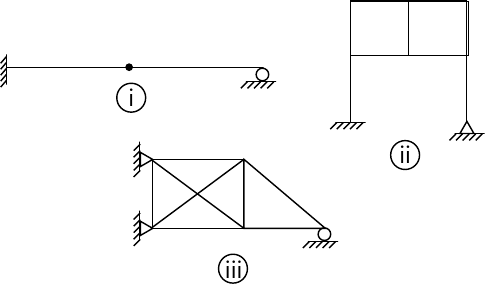3 M
1 (a) ii) Kinematic indeterminacy (neglecting axial deformation for flexural members)3 M
1 (b) Determine the horizontal displacement of joint D of the rigid jointed plane frame as shown in figure, due to change of temperature of member surfaces. Consider depth of all the member as 500mm. Take αt=12×10-6/°C.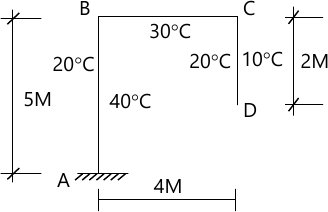10 M
1 (c) Determine the shape factor for the triangular section as shown in figure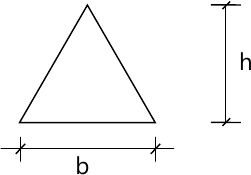4 M

2 (a) Analysis the rigid joined plane frame as shown in figure, using moment distribution Method. Draw BMD,SFD and elastic curve.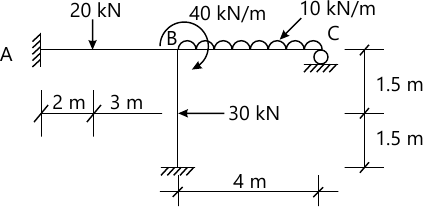10 M
2 (b) Analyse the beam by three moment theorem and Draw B.M.D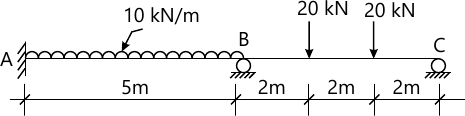10 M

3 (a) Analysis the rigid joined plane frame as shown in figure, using slope deflection method. Draw BMD,SFD and deflected shape.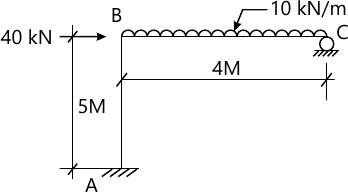12 M
3 (b) A two hinged parabolic arch of span-20 meter and rise 4 meter carries uniformly distributed load of 40kN/m on right half span find the reaction at the supports and deaw BMD.
8 M

4 (a) Analysis the rigid joined plane frame as shown in figure, using Flexibility Method. Draw BMD,SFD and deflected shape.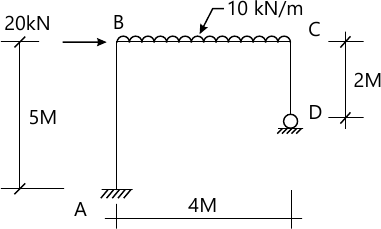12 M
4 (b) Analysis the beam by flexibility method and draw BMD.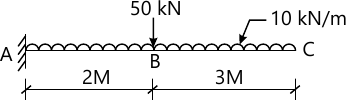8 M

5 (a) Analysis the rigid joined plane frame as shown in figure, using stiffness Method. Draw BMD, SFD and deflected shape.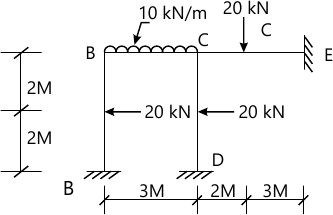12 M
5 (b) Calculate the plastic moment capacity required for the continuous beam with working load as shown in figure.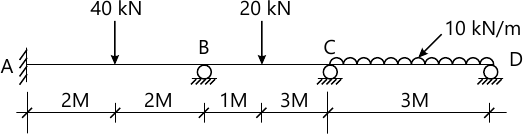8 M

6 (a) Analysis pin joined plane frame shown in figure and calculate the forces in all members. Take AE constant.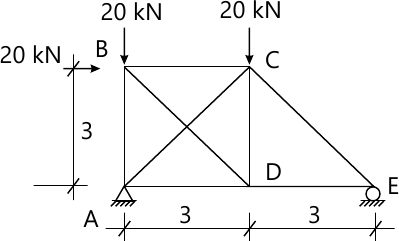12 M
6 (b) Analyze the beam as shown in figure using stiffness method.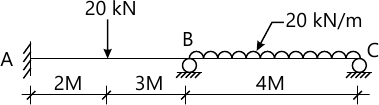8 M

More question papers from Structural Analysis - 2